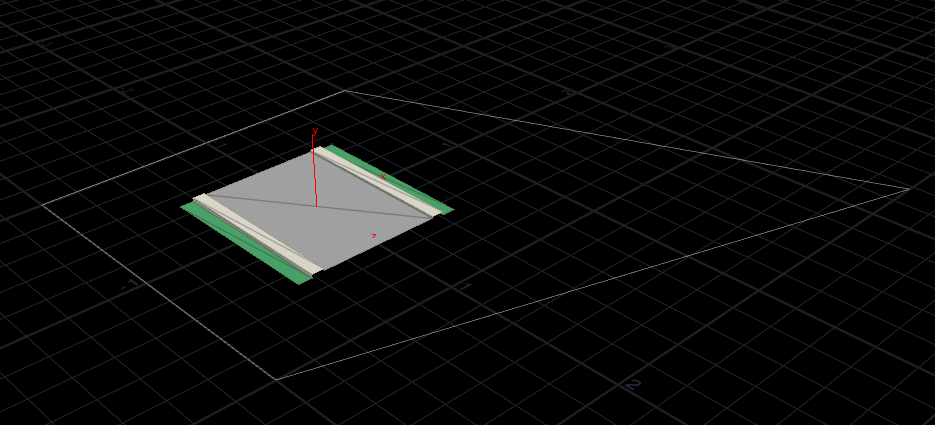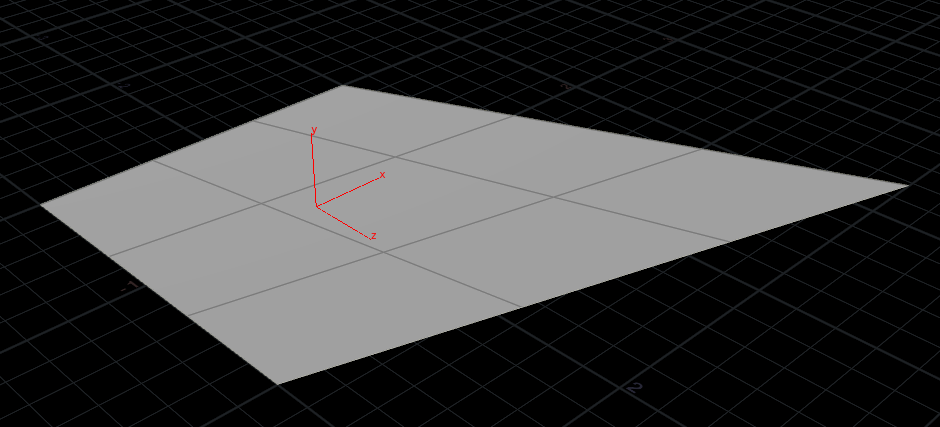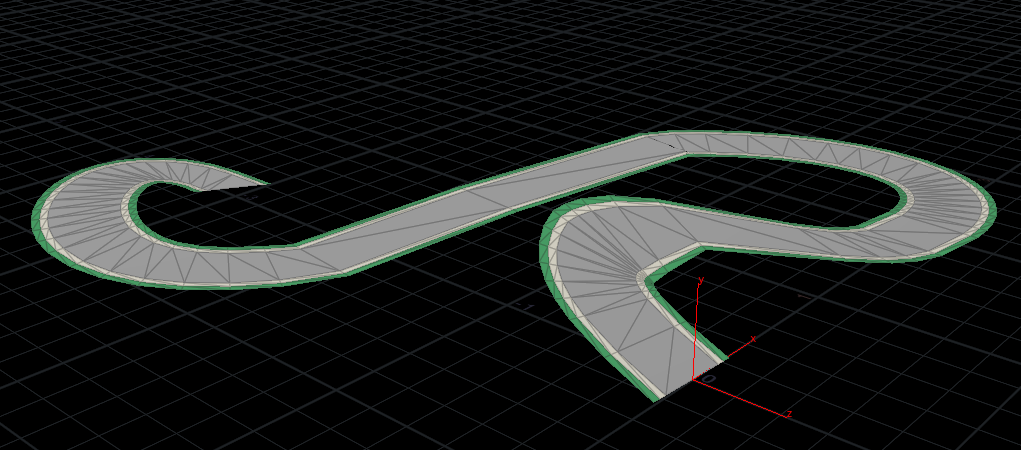Last week I finished with road geometry in Houdini. This alone is pretty bland; I want to be able to map geometry along the road. Specifically, I want to take an arbitrary input quad (four points that form a box) with whatever geometry inside the bounds, and map it to a target quad, preserving the relative positions of the inner geometry.This really smells like a change of basis to me. If I could express the initial corner positions, and the target positions as vector spaces, I would just need to find the single transformation matrix that would take any point from the intial space to the target space. Here’s where I found out how rusty my linear algebra is.

I sort of got somewhere by expressing a 2d space, with the basis vectors being along the diagonals of the quad. Then any point could be expressed as a linear combination of these orthogonal axes. Remapping is using the same linear combination with the new basis vectors. It worked for corners but lots of distortion around points off the basis vectors.Remapping a starting grid, intermediate points distorted

Bummer. This is really haunting me, because I’d really like to believe it’s possible and I’m setting it up wrong, but I also question if my example transformation - translating a single corner - is even a linear transformation.

At some point I threw out that idea and started to think that instead of diagonals I could take two parallel sides of the initial quad, and express each point as a percentage along each line, and then a percentage between those two points.

I got a hunch that maybe, like a bezier curve, I could identify a point’s relative position by computing the percentage along two of the parallel sides of the initial quad, getting two P0 and P1. Then I could compute the percentage between P0 and P1. Remapping would involve using the same percentages, but with those parallel sides transformed to the target. This stackexchange answer backed up my hunch, but generalized to more complex polygons.

Then I realized, textures do this everyday! UV mapping takes a 2d input texture (which is just a quad) and maps it to some target geometry. Here it’s a simpler problem, because my target isn’t some complex polygon; UV mapping should easily handle texturing a quad. Turns out, it’s the same technique, but much more formally described: Bilinear interpolation! Finally, the y/vertical axis just needs to be offset along the target quad normal, rather than the world up.Bilinear interpolation for gridOriginal input, repeatedly remapped along a poly strip.